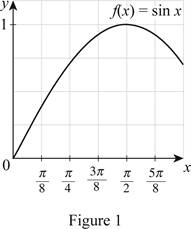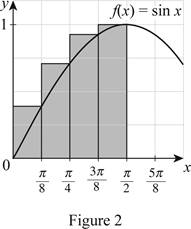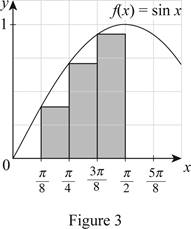# Whether the estimate is an underestimate or an overestimate using right endpoints.### Single Variable Calculus: Concepts...

4th Edition
James Stewart
Publisher: Cengage Learning
ISBN: 9781337687805### Single Variable Calculus: Concepts...

4th Edition
James Stewart
Publisher: Cengage Learning
ISBN: 9781337687805

#### Solutions

Chapter 5.1, Problem 4E

(a)

To determine

## To Analyze:Whether the estimate is an underestimate or an overestimate using right endpoints.

Expert Solution

The estimate is an overestimate for the function f(x)=sinx with four approximating rectangles and right endpoints.

### Explanation of Solution

Given information:

The curve function is f(x)=sinx.

The region lies between x=0 and x=π2. So, the limits are a=0 and b=π2.

The number of rectangles n=4.

Draw the graph for the function f(x)=sinx as shown below in Figure (1):Draw four approximating rectangles using right end points for the function f(x)=sinx as shown below in Figure (2):The expression to find the lower estimate of areas of n rectangles (Rn) is shown below:

Rn=f(x1)Δx+f(x2)Δx+...+f(xn)Δx (1)

Here, the right endpoint height of the first rectangle is f(x1), the width is Δx, the right endpoint height of the second rectangle is f(x2), and the right endpoint height of nth rectangle is f(xn).

Find the width (Δx) using the relation:

Δx=ban (2)

Here, the upper limit is b, the lower limit is a, and the number of rectangles is n.

Substitute π2 for b, 0 for a, and 4 for n in Equation (2).

Δx=π204=π8

Refer Figure (2).

Take the right endpoint height of the first rectangle f(x1) value as 0.38, the right endpoint height of the second rectangle f(x2) value as 0.7, the right endpoint height of the third rectangle f(x3) value as 0.93, and the right endpoint height of the fourth rectangle f(x4) value as 1.

Substitute 4 for n, 0.38for f(x1), π8 for Δx, 0.7 for f(x2), 0.93 for f(x3), and 1 for f(x4) in Equation (1).

R4=(0.38×π8)+(0.7×π8)+(0.93×π8)+(1×π8)=0.149+0.275+0.365+0.393=1.182.

Refer Figure (2).

The function curve as f(x)=sinx is the increasing curve in the interval between x=0 and x=π2. The intervals are represented as right end points.

Hence, R4 is an over estimate.

(b)

To determine

### To Analyze:Whether the estimate is an underestimate or an overestimate using right endpoints.

Expert Solution

The estimate is an underestimate for the function f(x)=sinx with four approximating rectangles and left endpoints.

### Explanation of Solution

Draw four approximating rectangles using left end points for the function f(x)=sinx as shown below in Figure (3):The expression to find the upper estimate of areas of 4 rectangles (L4) is shown below:

L4=R4+f(x0)Δxf(x3)Δx (3)

Here, the lower estimate using right endpoints for n=4 is R4, left endpoint height of left uppermost rectangle is f(x0), and the left endpoint height of the lowermost right rectangle is f(x3).

Refer Figure (3).

Take the left endpoint height of the uppermost left rectangle f(x0) value as 0.99 and the left endpoint height f(x3) value as 0.58.

Substitute 1.182 for R4, 0.38 for f(x0), π8 for Δx, and 0.94 for f(x3) in Equation (3).

L4=R4+f(x0)Δxf(x3)Δx=1.182+(0.38×π8)(0.94×π8)=1.182+0.1490.369=0.962.

Refer Figure (3).

The function curve as f(x)=sinx is increasing in the interval between x=0 and x=π2 and the intervals are represented as left end points.

Hence, L4 is an underestimate.

### Have a homework question?

Subscribe to bartleby learn! Ask subject matter experts 30 homework questions each month. Plus, you’ll have access to millions of step-by-step textbook answers!